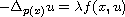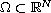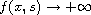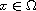Electron. J. Diff. Equ., Vol. 2014 (2014), No. 155, pp. 1-21.

### Existence of positive solutions for p(x)-Laplacian equations with a singular nonlinear term Jingjing Liu, Qihu Zhang, Chunshan Zhao

Abstract:
In this article, we study the existence of positive solutions for the p(x)-Laplacian Dirichlet problemin a bounded domain. The singular nonlinearity term f is allowed to be either, orasfor each. Our main results generalize the results in  from constant exponents to variable exponents. In particular, we give the asymptotic behavior of solutions of a simpler equation which is useful for finding supersolutions of differential equations with variable exponents, which is of independent interest.

Submitted July 2, 2013. Published July 7, 2014.
Math Subject Classifications: 35J25, 35J65, 35J70.
Key Words: p(x)-Laplacian; singular nonlinear term; sub-supersolution method.

Show me the PDF file (335 KB), TEX file, and other files for this article.Jingjing Liu College of Mathematics and Information Science Zhengzhou University of Light Industry Zhengzhou, Henan 450002, China email: jingjing830306@163.com Qihu Zhang (corresponding author) College of Mathematics and Information Science Zhengzhou University of Light Industry Zhengzhou, Henan 450002, China email:zhangqihu@yahoo.com, zhangqh1999@yahoo.com.cn Chunshan Zhao Department of Mathematical Sciences Georgia Southern University Statesboro, GA 30460, USA email: czhao@GeorgiaSouthern.edu